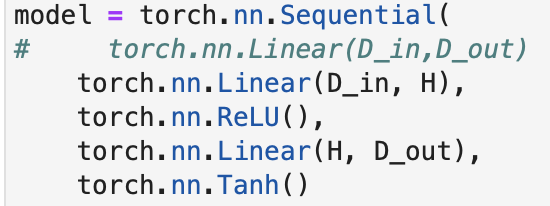I can’t figure out how to implement this operation with pytorch: \nabla \left( \nabla V_{\theta}^{\top}(s) \cdot \eta \right)V_{\theta} is an ANN that takes in a vector ‘s’, the gradient is the partial w.r.t. weights of the NN and \eta is a the same size as the network weights.

My main question: how to perform the second derivative in pytorch after taking the dot product with ‘\eta’?

I am using autograd.grad(out,in), with in= ANN weights, and the out is the output for some ‘s’. This provides me with the first gradient. But then once I dot the first derivative with ‘\eta’, I am no longer able to computer the gradient with autograd.grad() because there is no computational graph.

# -*- coding: utf-8 -*-
import torch
import math

dtype = torch.float
device = torch.device("cpu")

# N is batch size; D_in is input dimension;
# H is hidden dimension; D_out is output dimension.
N, D_in, H, D_out = 64, 3, 5, 2

# Create random Tensors to hold inputs and outputs
x = torch.randn(N, D_in, device=device)
y = torch.randn(N, D_out, device=device)

# Use the nn package to define our model as a sequence of layers. nn.Sequential
# is a Module which contains other Modules, and applies them in sequence to
# produce its output. Each Linear Module computes output from input using a
# linear function, and holds internal Tensors for its weight and bias.
model = torch.nn.Sequential(
torch.nn.Linear(D_in,D_out)
#     torch.nn.Linear(D_in, H),
#     torch.nn.ReLU(),
#     torch.nn.Linear(H, D_out),
)
model.to(device)
# The nn package also contains definitions of popular loss functions; in this
# case we will use Mean Squared Error (MSE) as our loss function.
loss_fn = torch.nn.MSELoss(reduction='sum')

learning_rate = 1e-4
for t in range(100):
# Forward pass: compute predicted y by passing x to the model. Module objects
# override the __call__ operator so you can call them like functions. When
# doing so you pass a Tensor of input data to the Module and it produces
# a Tensor of output data.
y_pred = model(x)

# Compute and print loss. We pass Tensors containing the predicted and true
# values of y, and the loss function returns a Tensor containing the
# loss.
loss = loss_fn(y_pred, y)
if t % 100 == 99:
print(t, loss.item())

# Zero the gradients before running the backward pass.

# Backward pass: compute gradient of the loss with respect to all the learnable
# parameters of the model. Internally, the parameters of each Module are stored
# in Tensors with requires_grad=True, so this call will compute gradients for
# all learnable parameters in the model.
loss.backward()
print(loss)

# Update the weights using gradient descent. Each parameter is a Tensor, so
# we can access its gradients like we did before.
for param in model.parameters():

outputs = model(torch.tensor([1.,2.5,3.5]))
eta =  [torch.rand(x.shape) for x in grad]
print(eta)
mult = sum([torch.dot(torch.flatten(x),torch.flatten(y)) for x,y in zip(grad,eta)])


You need to have create_graph=True when doing torch.autograd.grad for the first time so that the computation graph is built when computing grad.

I had updated the torch.dot() to requires_grad=True but it gives the error:
One of the differentiated Tensors appears to not have been used in the graph. Set allow_unused=True if this is the desired behavior.

I believe this is from the summation. Updated:

outputs = model(torch.tensor([1.,2.5,3.5]))
print(eta)
print(mult)


You are calling .item and also creating a new tensor on this line both of which break the graph.
You can do something like torch.sum(torch.stack([torch.dot(x.flatten(), y.flatten()) for x, y in zip(grad, eta)])) which should preserve the graph

I had tried with torch.stack() and it didn’t work either. I thought that maybe it is the list comprehension so I tried vectorizing the model resulting grad parameters, but that doesn’t work either. I am not sure if autograd.grad is retaining the graph because when I print it out there is no requires_grad=True attribute:

Whether param will still be in the backward graph of mult will depend on the specifics of the model. For example if we had a linear model, grad of outputs wrt to parameters, is just your model inputs * grad_output, i.e., its not a function of the weight.

But the custom model I defined here is an example of one that does work because the partial derivative of X * k**2 wrt k is still 2 * X * k

import torch
import torch.nn as nn

model_type = "custom"

class Model(nn.Module):
def __init__(self):
super(Model, self).__init__()
self.k = nn.Parameter(torch.rand(1))
def forward(self, X):
# the reason we need to clone here is because
# if an optimizer updates k in-place in no-grad mode it would increment the
# k's version counter, which could be an issue if k is saved for backward
return X * self.k.clone() ** 2

if model_type == "linear":
model = nn.Linear(10, 10)
elif model_type == "custom":
model = Model()

y_hat = model(X).sum()
eta = [torch.rand_like(t, requires_grad=True) for t in model.parameters()]